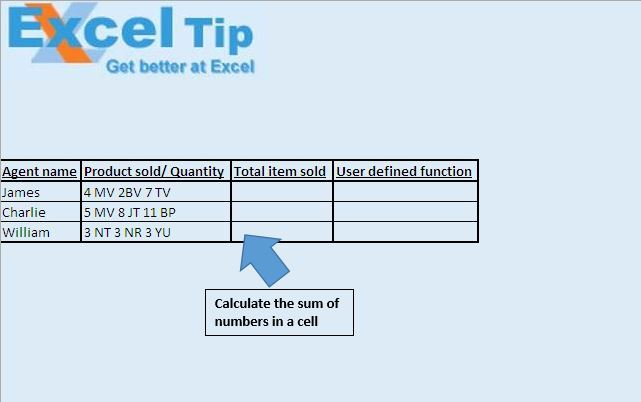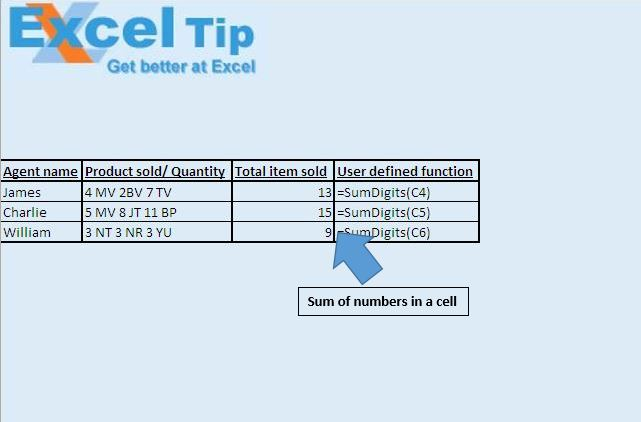# Sum the digits numbers in a cell using custom VBA function in Microsoft Excel

In this article, we will create a custom function to calculate the sum of digits in a cell.

Sample data for this example consists of sales data. Each agent has sold different product. In a cell we have count of item sold followed by the product name. We want to find the total item sold by each agent.We have created “SumDigits” function to calculate the sum of digits in the cell. This function takes range of cell as input and returns the sum of digits in the cell.Logic explanation

We have created custom function “SumDigits” to calculate the sum of digits in a cell. In this function, we find the length of string in the cell and then loop from first character to the last character in the string. Then we find and add the numeric values found in the string to get the sum of digits.

Code explanation

Mid(Number, i, 1)

Mid function is used to extract the sub-string from the main string. Above code will extract one character from the main string at a time.

IsNumeric(Value)

IsNumeric function is used to check whether the given value is numeric.

Val(Value)

Val function is used to convert the string to numeric value.

Please follow below for the code

```
Option Explicit

Function SumDigits(Number As Variant)

Dim i As Integer

For i = 1 To Len(Number)
If IsNumeric(Mid(Number, i, 1)) Then
SumDigits = SumDigits + Val(Mid(Number, i, 1))
End If
Next i

End Function

```

If you liked this blog, share it with your friends on Facebook. Also, you can follow us on Twitter and Facebook.

We would love to hear from you, do let us know how we can improve our work and make it better for you. Write to us at info@exceltip.com

## Comments

1.It works but I tried to multiply instead of summing but it doesn't work. Of course I changed the Sumdigits to Proddigits and changed the "+" to "*". What am I doing wrong and is it even possible to do it?

2.Another approach...

Function SumDigits(ByVal Number as Long) as long
' ExcelJuggler 16052015.....exceljuggler.org
Do while Number >= 1
SumDigits = SumDigits + Number Mod 10
Number = Int (Number / 10)
Loop
End Function

Terms and Conditions of use

The applications/code on this site are distributed as is and without warranties or liability. In no event shall the owner of the copyrights, or the authors of the applications/code be liable for any loss of profit, any problems or any damage resulting from the use or evaluation of the applications/code.